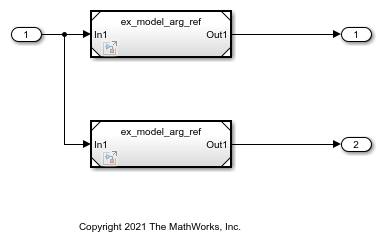# Group Multiple Model Arguments into a Single Structure

This example shows how to programmatically configure multiple instances of a referenced model to use different values for the same block parameter by using structures.

### Configure Referenced Model to Use Model Arguments Grouped into Structure

You can use structures to reduce the effort of maintenance when you want to add, rename, or delete arguments. With structures, the mathematical functionality of the models is the same.

To replace the parameter values with structures for `ex_model_arg_ref` and `ex_model_arg`, follow these steps.

Open model `ex_model_arg_ref`. This model represents a reusable algorithm.

```open_system('ex_model_arg_ref') ```Create a structure that contains one field for each of the parameter objects that exist in the `ex_model_arg_ref` workspace. Specify a value for each field.

```structForInst1.gain = 3.17; structForInst1.coeff = 1.05; ```

Store the structure in a `Simulink.Parameter` object.

```structForInst1Param = Simulink.Parameter(structForInst1); ```

Copy the `Simulink.Parameter` object into the `ex_model_arg_ref` model workspace. For this example, name the copy of the object `structArg`.

```modelWorkspace = get_param('ex_model_arg_ref','ModelWorkspace'); assignin(modelWorkspace,'structArg',copy(structForInst1Param)); ```

Configure `structArg` as the only model argument.

```set_param('ex_model_arg_ref','ParameterArgumentNames','structArg') ```

In the `ex_model_arg_ref` model, set the Gain parameter of the Gain block to `structArg.gain` and set the Numerator parameter of the Discrete Filter block to `structArg.coeff`.

```set_param('ex_model_arg_ref/Gain','Gain','structArg.gain') set_param('ex_model_arg_ref/Discrete Filter',... 'Numerator','structArg.coeff') ```

Copy the existing structure as `structForInst2Param`.

```structForInst2Param = copy(structForInst1Param); ```

Set the field values in the two structures to the same numbers that you used to set the model argument values in the Model blocks.

```structForInst1Param.Value.coeff = 0.98; structForInst1Param.Value.gain = 2.98; structForInst2Param.Value.coeff = 1.11; structForInst2Param.Value.gain = 3.34; ```

Open model `ex_model_arg`. This model represents a system model that uses multiple instances of the reusable algorithm.

```open_system('ex_model_arg') ```For model instance `Model`, set structArg to `structForInst1Param`. For model instance `Model1`, set structArg to `structForInst2Param`.

```instSpecParamsStruct = get_param('ex_model_arg/Model','InstanceParameters'); instSpecParamsStruct1 = get_param('ex_model_arg/Model1','InstanceParameters'); instSpecParamsStruct(1).Value = 'structForInst1Param'; instSpecParamsStruct1(1).Value = 'structForInst2Param'; set_param('ex_model_arg/Model','InstanceParameters',instSpecParamsStruct); set_param('ex_model_arg/Model1','InstanceParameters',instSpecParamsStruct1); ```

### Use Bus Object as Data Type of Structures

You can use a `Simulink.Bus` object as the data type of the structures. The bus object makes sure that the characteristics of the instance-specific structures, such as the names and order of fields, match the characteristics of the structure in the model workspace.

To set the data type of the structures to bus objects, follow these steps.

Use the `Simulink.Bus.createObject` function to create the bus object. The hierarchy of elements in the object matches the hierarchy of the structure fields. The default name of the object is `slBus1`.

```Simulink.Bus.createObject(structForInst1Param.Value); ```

Rename the bus object by copying it.

```myParamStructType = copy(slBus1); ```

Set the data type of the parameter objects in the base workspace by using the bus object.

```structForInst1Param.DataType = 'Bus: myParamStructType'; structForInst2Param.DataType = 'Bus: myParamStructType'; ```

For the `structArg` object, set `DataType` to `Bus: myParamStructType`.

```temp = getVariable(modelWorkspace,'structArg'); temp = copy(temp); temp.DataType = 'Bus: myParamStructType'; assignin(modelWorkspace,'structArg',copy(temp)); close_system('ex_model_arg_ref',0) close_system('ex_model_arg',0) ```